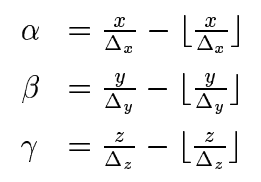Why itk::BSpineTransform add two extra control points to the upper bound?

(Alireza ) #1

I have a question regarding the extra control points that gets added to the grid for the B-spline transformation.
I fully understand the one control point that gets added to the lower bound, but I don’t get why two control points gets added to the upper bound of the grid? I expected to see only one control points get added to the upper bound too.

Let me explain more:

we have (from paper 1 and paper2)Let’s assume a 2D scenario (so no k index) in which we have couple of sampling points (the blue dots).

Based on the formula above the support control points (i,j indexes) are circled for Sample (A) and Sample (B). In both cases there is no need for the 2 added control points to the upper bound of the space. Only with 1 extra rows (added to the top and right hand side) the support can be achieved.

Am i missing something? Why does ITK add two extra control points to the upper bound instead of just 1 ?

I’m seeing the 3D slicer tutorial/documentation that only 1 row/column is added
https://www.slicer.org/wiki/Documentation/4.10/FAQ#What.27s_the_BSpline_Grid_Size.3F

1 Like
(Andras Lasso) #2

In the current implementation, each displacement is always computed from one control point below and two control points above, on each axis.

If you wanted to just use one row of control points below and above, then somewhere in the middle of the field you would need to switch from using “1 below+2 above” to using “2 below, 1 above” interpolation. This would at least complicate the implementation, but maybe it would even lead to interpolation artifacts.

2 Likes
(Matt McCormick) #3

It is also worth noting that the two additional control points occurs with a third order b-spline, which is the default in ITK. As noted in the itk::BSplineTransform documentation:

number of control points = mesh size + spline order

1 Like
(Alireza ) #4